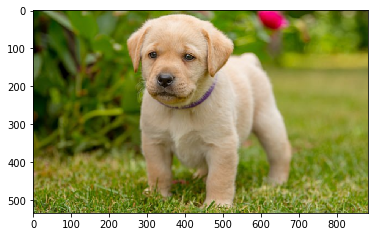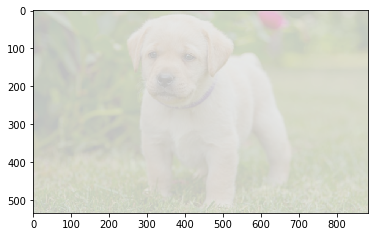# Mahotas – Image Stretch RGB

In this article we will see how we can do stretching for RGB image in mahotas. Contrast stretching (often called normalization) is a simple image enhancement technique that attempts to improve the contrast in an image by `stretching’ the range of intensity values it contains to span a desired range of values, e.g. the the full range of pixel values that the image type concerned allows. RGB stretching is ariation of stretch() function that works per-channel on an RGB image

In this tutorial we will use “lena” image, below is the command to load it.

`mahotas.demos.load('lena')`

Below is the lena imageIn order to do this we will use ` mahotas.regmin` method

Syntax : mahotas.stretch_rgb(img)

Argument : It takes image object as argument

Return : It returns image object

Below is the implementation

 `# importing required libraries ` `import` `mahotas ` `import` `mahotas.demos ` `from` `pylab ``import` `gray, imshow, show ` `import` `numpy as np ` `import` `matplotlib.pyplot as plt ` `   `  `# loading iamge ` `img ``=` `mahotas.demos.load(``'lena'``) ` `   `  `print``(``"Image"``) ` `   `  `# showing image ` `imshow(img) ` `show() ` ` `  `# strecting rgb image ` `new_img ``=` `mahotas.stretch_rgb(img) ` `  `  ` `  `# Stretched image ` `print``(``"Stretched Image"``) ` `imshow(new_img) ` `show() `

Output :

`Image``Stretched Image`Another example

 `# importing required libraries ` `import` `mahotas ` `import` `numpy as np ` `from` `pylab ``import` `gray, imshow, show ` `import` `os ` `import` `matplotlib.pyplot as plt ` `  `  `# loading iamge ` `img ``=` `mahotas.imread(``'dog_image.png'``) ` ` `  `   `  `print``(``"Image"``) ` `   `  `# showing image ` `imshow(img) ` `show() ` ` `  `# strecting image ` `new_img ``=` `mahotas.stretch_rgb(img) ` `  `  ` `  `# Stretched image ` `print``(``"Stretched Image"``) ` `imshow(new_img) ` `show() `

Output :

`Image``Stretched Image`My Personal Notes arrow_drop_upCheck out this Author's contributed articles.

If you like GeeksforGeeks and would like to contribute, you can also write an article using contribute.geeksforgeeks.org or mail your article to contribute@geeksforgeeks.org. See your article appearing on the GeeksforGeeks main page and help other Geeks.

Please Improve this article if you find anything incorrect by clicking on the "Improve Article" button below.

Article Tags :

Be the First to upvote.

Please write to us at contribute@geeksforgeeks.org to report any issue with the above content.